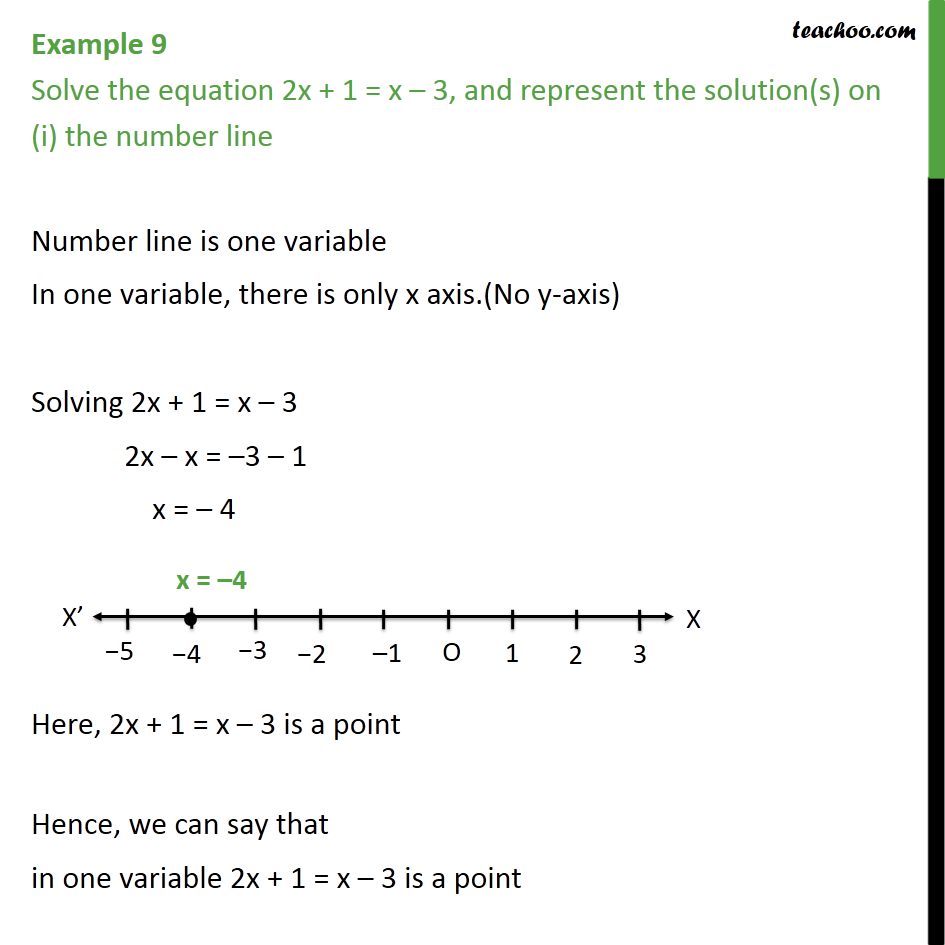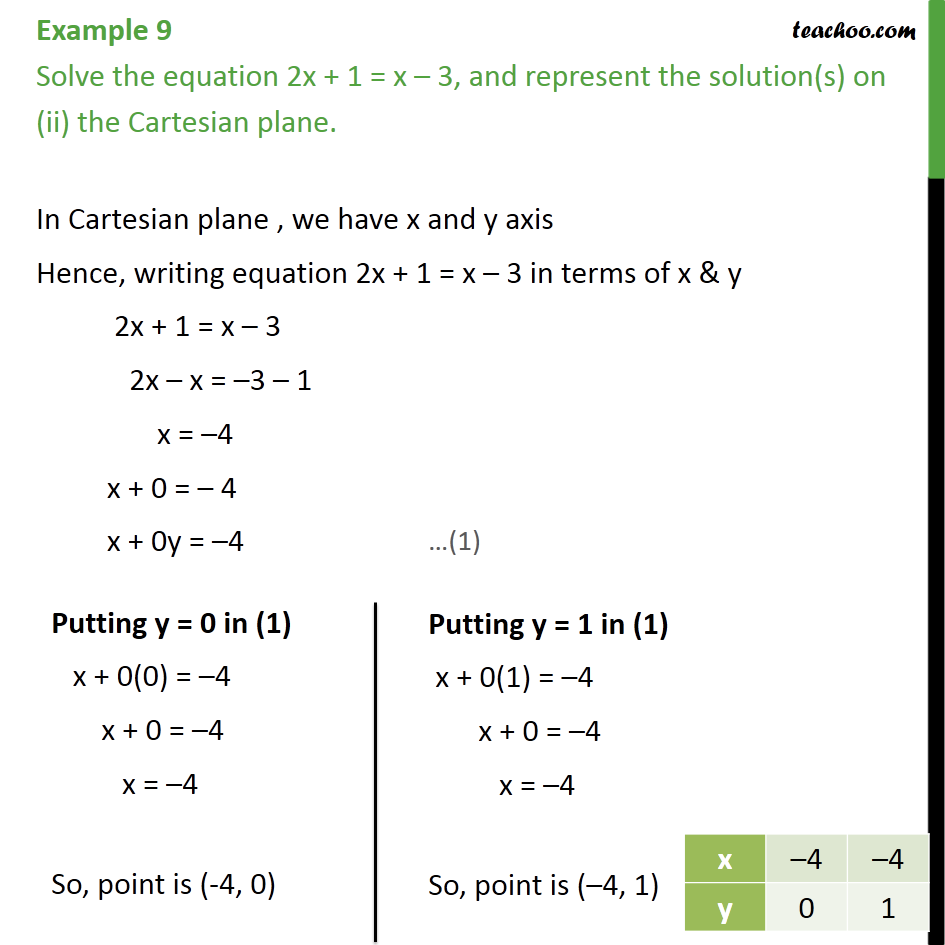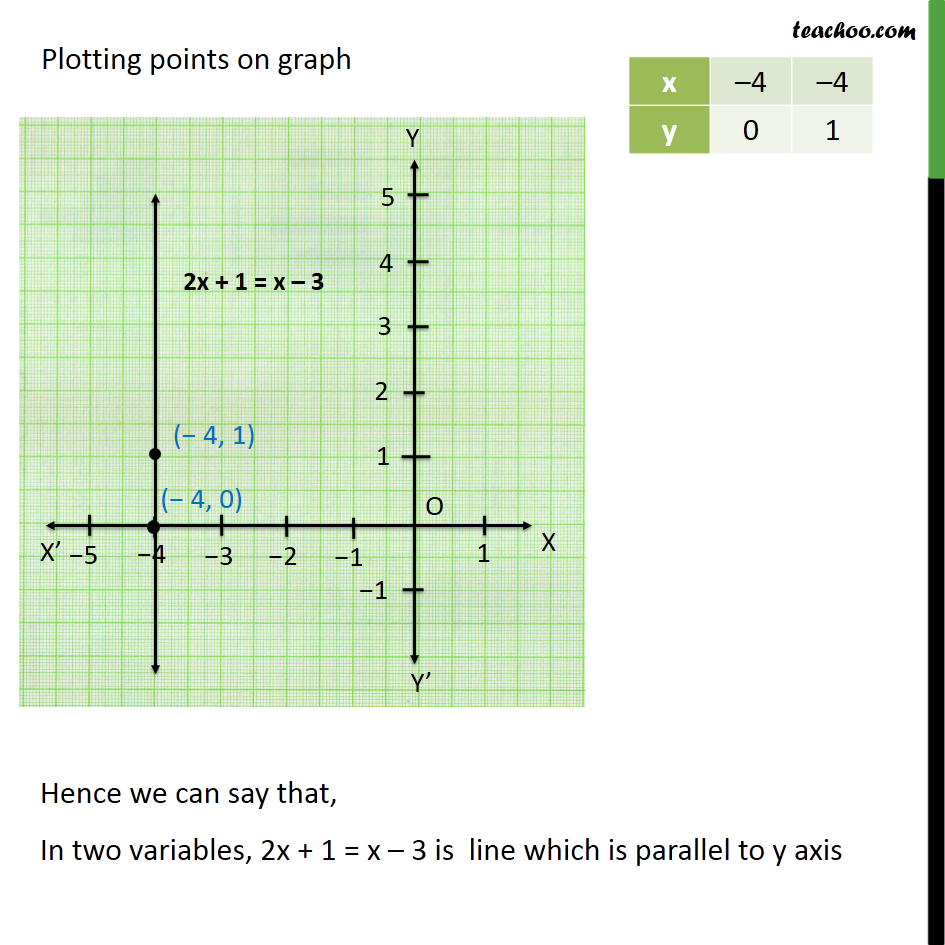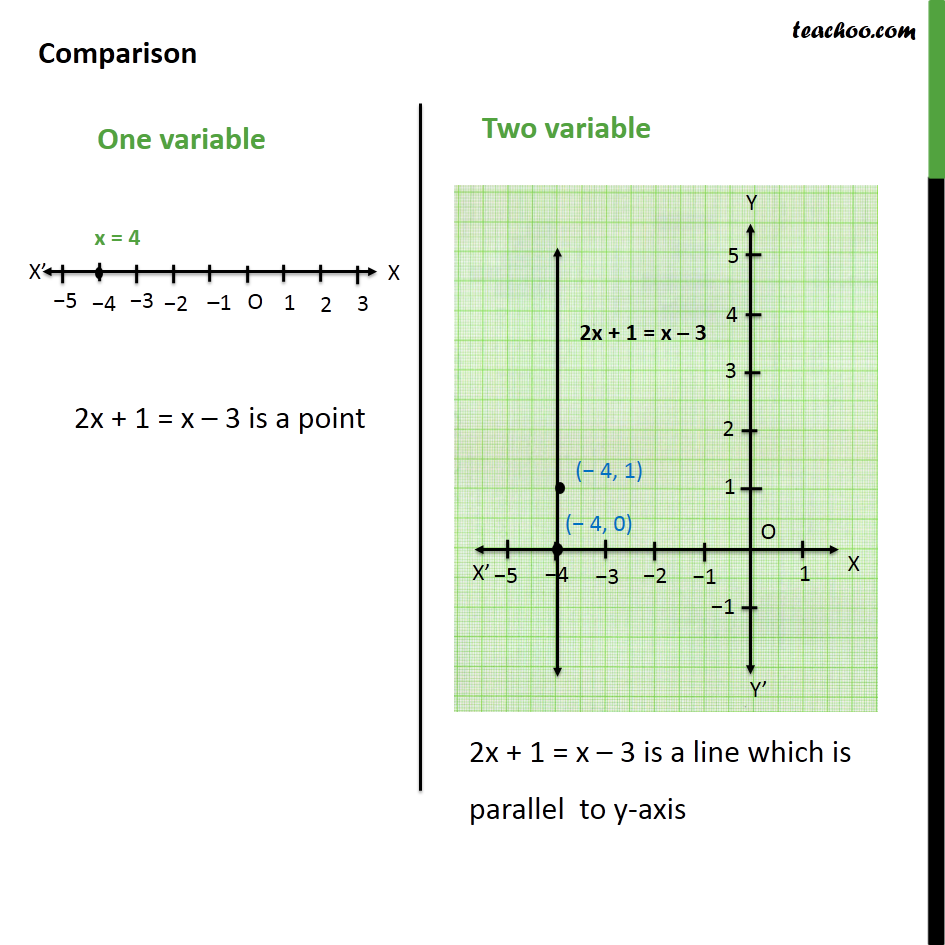Subscribe to our Youtube Channel - https://you.tube/teachoo

1. Class 9
2. Important Questions for Exam - Class 9
3. Chapter 4 Class 9 Linear Equations in Two Variables

Transcript

Example 9 Solve the equation 2x + 1 = x – 3, and represent the solution(s) on (i) the number line Number line is one variable In one variable, there is only x axis.(No y-axis) Solving 2x + 1 = x – 3 2x – x = –3 – 1 x = – 4 Here, 2x + 1 = x – 3 is a point Hence, we can say that in one variable 2x + 1 = x – 3 is a point Example 9 Solve the equation 2x + 1 = x – 3, and represent the solution(s) on (ii) the Cartesian plane. In Cartesian plane , we have x and y axis Hence, writing equation 2x + 1 = x – 3 in terms of x & y 2x + 1 = x – 3 2x – x = –3 – 1 x = –4 x + 0 = – 4 x + 0y = –4 Plotting points on graph Comparison

Chapter 4 Class 9 Linear Equations in Two Variables

Class 9
Important Questions for Exam - Class 9